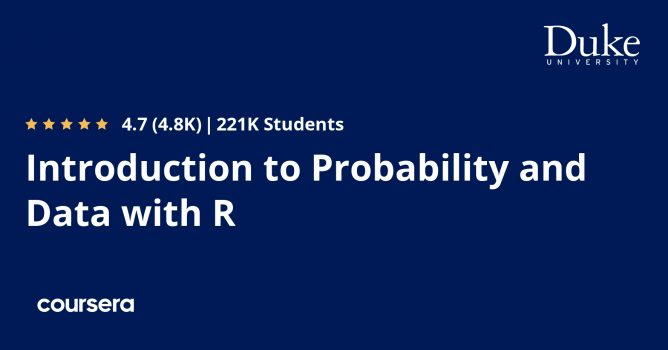# Introduction to Probability and Data with RIntroduction to Probability and Data with R It's a Free Course. Enroll it before the instructor makes it to the paid course.

#### What you will learn from the Introduction to Probability and Data with R

• Explore data via numerical summaries and visualizations
• Types of sampling methods
• Learn about rules of probability and commonly used probability distributions
• Explore Categorical Variables
• Visualise Numerical Data
• Probability & conditional probability
• Work with the Normal Distribution
• Binomial Distribution
• Work with the Binomial Distribution

Course Instructor Mine Çetinkaya-Rundel & Offered by Duke University from Coursera

Course Duration: 14 hours to complete

Course is for Beginner Level

#### Can I download Introduction to Probability and Data with R course?